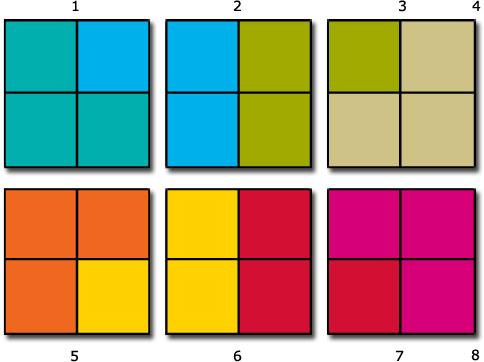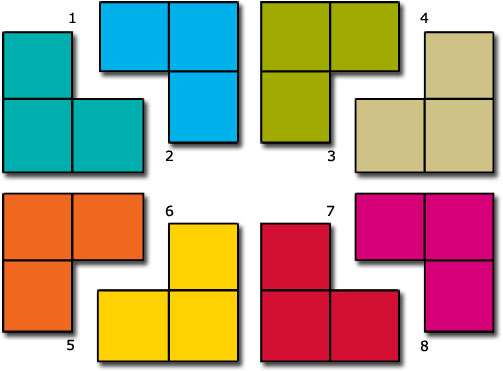Science, Maths & Technology

### Become an OU studentNumbers, units and arithmetic

Start this free course now. Just create an account and sign in. Enrol and complete the course for a free statement of participation or digital badge if available.

# 3.13 More division with fractions

How about other fractions? What is 6 ÷? This means how manyare there in 6? Consider six squares, divided into quarters, and count how many groups of three quarters there are.

## Activity 51Three quarters is 3 ×? So dividing byis the same as dividing by 3 and by. Dividing byis multiplying by 4. So dividing byis the same as dividing by 3 and multiplying by 4, which is multiplying by. So 6 ÷= 6 ×== 8.

The general rule for dividing by a fraction can be stated as follows.

To divide by a fraction, turn the fraction upside down and multiply.

Does this also work for dividing by? Yes, because multiplying by n is the same as multiplying by. It also works for division of whole numbers: dividing by 4 (=) is the same as multiplying by.

## Example 23

Evaluate:

•• Using the rule above,

•• This is

•(divide top and bottom by 3).

• Alternatively divide top and bottom by 3 by cancelling by 3 to give

•• So.

Turning a fraction upside down is called finding its reciprocal.

The reciprocal ofis. The reciprocal ofis, i.e. 6.

So an alternative formulation of the rule for dividing by a number can be stated as follows.

Division by a number is the same as multiplication by its reciprocal.